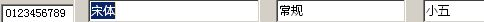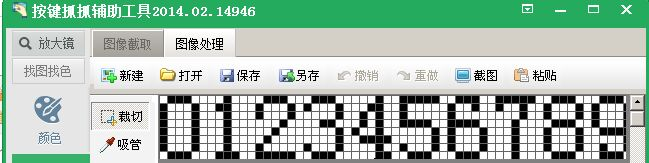1#上一篇院刊我们已经介绍了几种常见的验证码形式，相信大家对验证码都有了一定的了解，这期我们就去了解下一些简单验证码的识别的思路和流程，在破解验证码中需要用到的知识一般是 像素、线、面等基本2维图形元素的处理和色差分析，这方面小编也是刚刚接触，所以今天就重点介绍下在不使用第三方插件的情况下最原始的依靠像素识别数字、字母的方法——点阵识别法

[tr=rgb(154, 205, 50)]
 什么是点阵？

[tr=rgb(154, 205, 50)]
 如何用点阵识别法识别数字？0就可以看成0111010001100011000110001100011000101110
1就可以看成0010001100001000010000100001000010001110

1. '赋值下十个特征码，开头代码执行一次就可以了
2. NO0="0111010001100011000110001100011000101110"
3. NO1="0010001100001000010000100001000010001110"
4. NO2="0111010001100010001000100010001000011111"
5. NO3="0111010001000010011000001000011000101110"
6. NO4="0001000110010100101010010011110001000011"
7. NO5="1111110000100001111000001000011000101110"
8. NO6="0111010010100001111010001100011000101110"
9. NO7="1111110010000100010000100001000010000100"
10. NO8="0111010001100010111010001100011000101110"
11. NO9="0111010001100011000101111000010100101110"
12. MsgBox GetShu(283, 258, 6, 4, "000000")
13. '以下是写好的函数模块，写好基本就不用修改了，需要的时候调用执行即可
14. Function GetShu(x, y, z, n, col)'传入要识别的数字前台左上角坐标xy，俩数间间距z，数量n，字体颜色col
15. GetShu = ""
16. Dim code, temp_x, i_x, i_y, num
17. For temp_x = 0 To n - 1
18. code = ""
19. For i_y = 0 To 7
20. For i_x = 0 To 4
21. If GetPixelColor(x + i_x + temp_x * z, y + i_y) = col Then
22. code = code & "1"
23. Else
24. code = code & "0"
25. End If
26. Next
27. Next
28. If code = NO0 Then
29. num="0"
30. ElseIf code = NO1 Then
31. num="1"
32. ElseIf code = NO2 Then
33. num="2"
34. ElseIf code = NO3 Then
35. num="3"
36. ElseIf code = NO4 Then
37. num="4"
38. ElseIf code = NO5 Then
39. num="5"
40. ElseIf code = NO6 Then
41. num="6"
42. ElseIf code = NO7 Then
43. num="7"
44. ElseIf code = NO8 Then
45. num="8"
46. ElseIf code = NO9 Then
47. num = "9"
48. Else
49. num = "?"
50. End If
51. GetShu = GetShu & num
52. Next
53. End Function

[tr=rgb(154, 205, 50)]
 点阵法的局限性

1.缺点很明显，必须是字体清晰，字与字间距一致的才可正常识别
2.字体不同，粗细不同，点阵特征都不一样，没有哪个点阵特征码是可以通用的。
3.识别数字或字母的方法和思路有很多，并不是单一的点阵（一点一点的）识别，，每种识别方法都各有利弊。

【院刊】-【201507期】千奇百怪的验证码
【院刊】-【201504期】妹子“卖身”为哪般？验证码真的这么难识别？

showtopic.aspx?topicid=549014&forumpage=1&page=1

[tr=rgb(143, 188, 143)]
 按键精灵实战班开始报名啦！ 腾讯课堂视频课程 1、时间：7月1日——7月25日接受报名2、时长：每周二、周四、周六3、地点：实战班4、项目准备：安装按键精灵2014，安装镇魔曲、按键精灵会员卡（报名会提供3个月）。5、关于项目课程的安排：周一至周天白天可自行学习与实践，学院会提供为学员准备助教与老师进行解答，周二、周四、周六晚上8点开始授课，请各位学员到场学习。6、学费费用：512（人民币）7、本次实战课程全国招募66人，名额有限。 【招生对象】：零基础用户，快速学会按键精灵安卓版【招生人数】：70人【报名时间】：3/18——4/6【学费】：128RMB【学习日期】：1个月（2015.4.6-2015.4.27）【上课时间】：周1、周3、周5 19：30--21：30【上课导师】：梦幻小飞侠【实战游戏】：[天天富翁]、[三国之刃]、[梦幻西游]、[天 龙 八 部] 点我进入按键学院报名啦招生细节进群咨询招生办主任